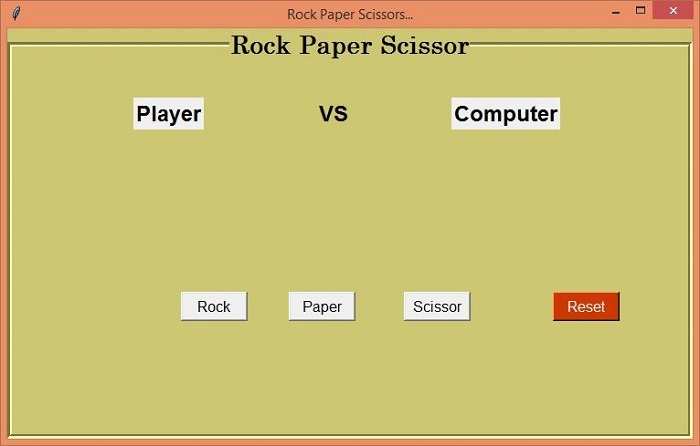# Rock Paper and Scissor Game Using Tkinter

Tkinter is one of the Python-based libraries used to create and develop Desktop User interfaces and applications. Using the Tkinter library and its packages, we will create a Rock Paper Scissor Game Application. The game can be played between two people using hand gestures. The condition for winning the game is,

• If player A gets Paper and Player B gets scissors, then Scissor wins.

• If player A gets Paper and Player B gets Rock, then Paper wins.

Similarly,

• If player A gets Rock and Player B gets scissors, then Rock wins.

Following these game conditions, we will first create the GUI for the game user interface. The user can play with the device as the opponent itself. Our GUI application will have the following features,

• The application will have a Title following the User and Opponent.

• To denote the hand gestures for Rock, Paper, and scissors, we will create three buttons for each.

• Once the game gets started, it will show the final winner on the application window.

• Reset button to reset the Game.

## Example

#import required libraries
from tkinter import *
from tkinter import ttk
import random

#Create an instance of tkinter frame
win= Tk()

#Set the geometry of the window
win.geometry("750x450")

#Set the title of the window
win.title("Rock Paper Scissors...")

#Default value for Computer
computer_options = {
"0":"Rock",
"1":"Paper",
"2":"Scissor"
}

#Disable all the Buttons after first Match
def button_disable():
b1.config(state= "disabled")
b2.config(state= "disabled")
b3.config(state= "disabled")

#Define function for Rock
def isrock():
value = computer_options[str(random.randint(0,2))]
if value == "Rock":
match_result = "Match Draw"
elif value=="Scissor":
match_result = "Wohoo! You Won"
else:
match_result = "Computer Win"
label.config(text = match_result)
l1.config(text = "Rock")
l3.config(text =value)
button_disable()

#Function for Paper
def ispaper():
value = computer_options[str(random.randint(0, 2))]
if value == "Paper":
match_result = "Match Draw"
elif value=="Scissor":
match_result = "Computer Win"
else:
match_result = "Amazingg..You won"
label.config(text = match_result)
l1.config(text = "Paper")
l3.config(text = value)
button_disable()

#Function for Scissor
def isscissor():
value = computer_options[str(random.randint(0,2))]
if value == "Rock":
match_result = "Computer Win"
elif value == "Scissor":
match_result = "Match Draw"
else:
match_result = "You Win... :D"
label.config(text = match_result)
l1.config(text = "Scissor")
l3.config(text = value)
button_disable()

#Reset the Game
def reset():
b1.config(state= "active")
b2.config(state= "active")
b3.config(state= "active")
l1.config(text = "Player")
l3.config(text = "Computer")
label.config(text = "")

#Create a LabelFrame
labelframe= LabelFrame(win, text= "Rock Paper Scissor", font= ('Century 20 bold'),labelanchor= "n",bd=5,bg= "khaki3",width= 600, height= 450, cursor= "target")
labelframe.pack(expand= True, fill= BOTH)

#Label for Player
l1= Label(labelframe, text="Player", font= ('Helvetica 18 bold'))
l1.place(relx= .18, rely= .1)

#Label for VS
l2= Label(labelframe, text="VS", font= ('Helvetica 18 bold'), bg="khaki3")
l2.place(relx= .45, rely= .1)

#Label for Computer
l3= Label(labelframe, text="Computer", font= ('Helvetica 18 bold'))
l3.place(relx= .65, rely= .1)

#Create a label to display the Conditions
label= Label(labelframe, text="", font=('Coveat', 25,'bold'), bg= "khaki3")

#Create Button Set for Rock, Paper and Scissor
b1= Button(labelframe, text= "Rock", font= 10, width= 7, command= isrock)
b1.place(relx=.25, rely= .62)
b2= Button(labelframe, text= "Paper", font= 10, width= 7 ,command= ispaper)
b2.place(relx= .41,rely= .62)
b3= Button(labelframe, text= "Scissor", font= 10, width= 7, command= isscissor)
b3.place(relx= .58, rely= .62)

#Button to reset the Game
reset= Button(labelframe, text= "Reset",bg= "OrangeRed3", fg= "white",width= 7, font= 10, command= reset)
reset.place(relx= .8, rely= .62)
win.mainloop()

## Output

Running the above code will display the GUI of the game. We can now play by clicking any of the buttons "Rock," "Paper," or "Scissor."